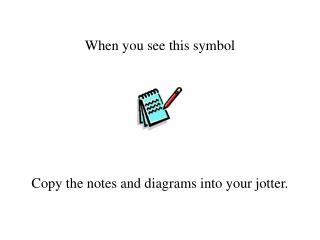DownloadDownload PresentationWhen you see this symbol

# When you see this symbol

Download Presentation## When you see this symbol

- - - - - - - - - - - - - - - - - - - - - - - - - - - E N D - - - - - - - - - - - - - - - - - - - - - - - - - - -
##### Presentation Transcript

1. When you see this symbol Copy the notes and diagrams into your jotter.

2. The area under a curve Let us first consider the irregular shape shown opposite. How can we find the area A of this shape?

3. The area under a curve We can find an approximation by placing a grid of squares over it. By counting squares, A > 33 and A < 60 i.e. 33 < A < 60

4. The area under a curve By taking a finer ‘mesh’ of squares we could obtain a better approximation for A. We now study another way of approximating to A, using rectangles, in which A can be found by a limit process.

5. The area under a curve The diagram shows part of the curve y = f(x) from x = a to x = b. A We will find an expression for the area A bounded by the curve, the x-axis, and the lines x = a and x = b.

6. Δx The area under a curve The interval [a,b] is divided into n sections of equal width, Δx. A n rectangles are then drawn to approximate the area A under the curve.

7. f(x1) Δx1 The area under a curve Dashed lines represent the height of each rectangle. The position of each line is given by an x-coordinate, xn. The first rectangle has height f(x1) x1, x2 ,x3, x4 , x5, x6 and breadthΔx1. Thus the area of the first rectangle = f(x1).Δx1

8. The area under a curve An approximation for the area under the curve, between x = a to x = b, can be found by summing the areas of the rectangles. A =f(x1).Δx1 + f(x2).Δx2 + f(x3).Δx3 + f(x4).Δx4 + f(x5).Δx5 + f(x6).Δx6

9. The area under a curve Using the Greek letter Σ (sigma) to denote ‘the sum of’, we have For any number n rectangles, we then have

10. The area under a curve In order to emphasise that the sum extends over the interval [a,b], we often write the sum as

11. The area under a curve By increasing the number n rectangles, we decrease their breadth Δx. As Δx gets increasingly smaller we say it ‘tends to zero’, i.e. Δx 0. Remember, we met limits before with Differentiation So we define

12. The form The area under a curve was simplified into the form that we are familiar with today This reads ‘the area A is equal to the integral of f(x) from a to b’.

13. We have seen the integration symbol before in connection with anti-differentiation, but we have not yet connected finding the area under a curve with the process of integration. The area under a curve We have derived a method for finding the area under a curve and a formal notation

14. The area under a curve Let us remind ourselves of where we started. Can we apply this method to calculate the area under a curve?

15. f(x) f(x+h) h x x+h A(x) A(x+h) x+h The area under a curve Consider a strip under the curve h wide. The inner rectangle has area h f(x). The outer rectangle has area h f(x+h). The actual area is given by A(x+h) – A(x).

16. f(x) f(x+h) h x x+h A(x) The area under a curve Comparing areas, h f(x)  A(x+h) – A(x) h f(x+h)

17. by the definition of integration. The area under a curve h f(x)  A(x+h) – A(x) h f(x+h) So f(x) = A’(x),by the definition of a derived function

18. The area under a curve In conclusion, the area A bounded by the x-axis, the lines x = a and x = b and the curve y = f(x) is denoted by,